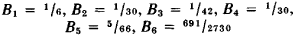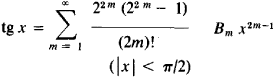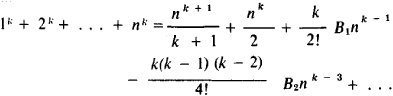# Bernoulli Numbers

The following article is from The Great Soviet Encyclopedia (1979). It might be outdated or ideologically biased.

## Bernoulli Numbers

a special sequence of rational numbers which figures in various problems of mathematical analysis and the theory of numbers. The values of the first six Bernoulli numbers areIn mathematical analysis, Bernoulli numbers appear as the coefficients of expansion of certain elementary functions in power series—for example,The Euler-Maclaurin summation formula is one of the most important formulas in which Bernoulli numbers are encountered. The sums of many series and the values of improper integrals are expressed in terms of Bernoulli numbers. Bernoulli numbers first appeared in the posthumous work of Jakob Bernoulli (1713) in connection with the calculation of the sum of identical powers of natural numbers. He proved thatRecurrence formulas that permit the sequential calculation of Bernoulli numbers, as well as explicit formulas (which have a rather complex form), are known for Bernoulli numbers.

There is great interest in the theoretic-numerical properties of the Bernoulli numbers. In 1850 the German mathematician E. Kummer established that Fermat’s equation xp + yp = zp is not solved in integers x, y, and z which are not zero unless a prime number p > 2 divides the numerators of the Bernoulli numbers B1, B2, . . ., B(p - 3)/2. Often (-1)m-1 B2m (m = 1, 2,. . .) is written instead of Bm to designate Bernoulli numbers; furthermore, it is assumed that

B0 = 1, B1 = -½, B3 = B5 = B7 = . . . = 0

### REFERENCES

Chistiakov, I. I. Bernullievye chisla. Moscow, 1895.
Kudriavtsev, V. A. Summirovanie stepenei chisel natural’nogo riada i chisla Bernulli. Moscow-Leningrad, 1936.
Whittaker, E. T., and G. N. Watson. Kurs sovremennogo analiza, 2nd ed., part 1. Moscow, 1963. (Translated from English.)
Landau, E. Vorlesungenüber Zahlentheorie, vol. 3. New York, 1927.

S. B. STECHKIN

References in periodicals archive ?
In , Arakawa and Kaneko used analytic continuation of [zeta]([s.sub.1], ..., [s.sub.d]) as a function of one variable [s.sub.d] when [s.sub.1], ..., [s.sub.d-1] are positive integers and discussed the relation among generalized Bernoulli numbers. For a general d, Zhao  proved the analytic continuation of [zeta]([s.sub.1], ..., [s.sub.d]) as a function of d variables using the theory of generalized function and Akiyama, Egami and Tanigawa  proved the same result by applying the classical Euler-Maclaurin formula to the index of the summation [n.sub.d].
where [[alpha].sub.i], i = 0, 1, ..., m are the Bernoulli numbers. Thus, the first four such polynomials, respectively, are
For the second edition he has corrected errors; clarified some confusion; and added new problems on Bernoulli numbers, metric spaces, and differential equations.
where [f.sup.(n)](s) denotes the n-order derivative of f(s), [B.sub.2k] is Bernoulli numbers.
269-270]) contains the fundamental Bernoulli's formula which expresses the sum [S.sub.r](n) = [[summation].sup.n-1.sub.i=1] [i.sup.r] (r = 0, 1, 2, ...) as a (r+ 1)th-degree polynomial function on n whose coefficients involve Bernoulli numbers. Namely, the celebrated Bernoulli's
with an effective expansion parameter g = [(eB/[m.sup.2]).sup.2], and coefficients [c.sup.(1).sub.n] that can be written in terms of the Bernoulli numbers [B.sub.n]:
represent the Bernoulli numbers 1, -1/2, 1/6, 0, -1/30, 0, 1/42, 0, -1/30, 0, ..., with [B.sub.2k+1] = 0 for k [greater than or equal to] 1.
It is well known that the Laurent series expansion of this function we can write using the Bernoulli numbers [B.sub.2k], namely,
They included a way to calculate Bernoulli numbers (a mathematical sequence of numbers) using the machine.
for n [greater than or equal to] 0, where [B.sub.k] are the Bernoulli numbers.
latter's papers on the Bernoulli numbers [Gen52, Gen86].
Let [B.sub.k](t), k[greater than or equal to]0 be the Bernoulli polynomials, and [B.sub.k] = [B.sub.k](0), k[greater than or equal to]0, the Bernoulli numbers. The first few Bernoulli polynomials are

Site: Follow: Share:
Open / Close